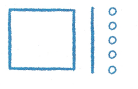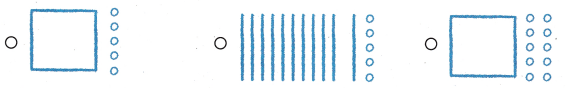# Texas Go Math Grade 1 Lesson 1.8 Answer Key Expanded Form

Refer to our Texas Go Math Grade 1 Answer Key Pdf to score good marks in the exams. Test yourself by practicing the problems from Texas Go Math Grade 1 Lesson 1.8 Answer Key Expanded Form.

## Texas Go Math Grade 1 Lesson 1.8 Answer Key Expanded Form

Essential Question
How can you write 112 in expanded form?

Explore
Use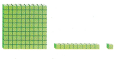to model the problem.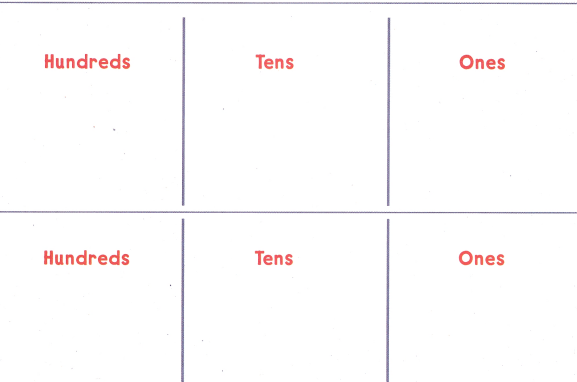Explanation:
Total number is blocks are 112
So we can write as 1 hundred 1 tens and 2 ones  or 11 tens and 2 ones

For The Teacher

• Ask children to use base-ten blocks to show 112 two different ways

Math Talk
Mathematical Processes

How did you decide how many hundreds, tens, and ones are in 112? Explain.
112 is a three-digit number.
The 1 in 112means 1 hundred.
The 1 in 112 means 1 ten.
The  2 in 112 means 2 ones.

Model and Draw
115 is a three-digit number.
The 1 in 115 means 1 hundred.
The 1 in 115 means 1 ten.
The 5 in 115 means 5 ones.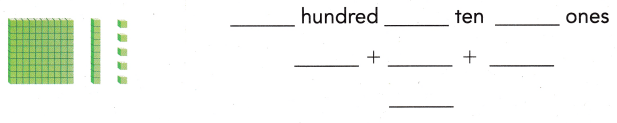Explanation:
Total number is blocks are 115
So we can write as 1 hundred 1 tens and 5 ones

Share and Show

Use the model. Write the number three different ways.

Question 1.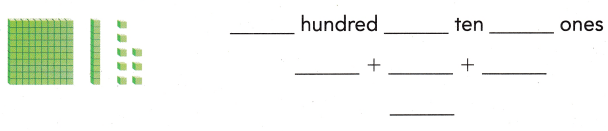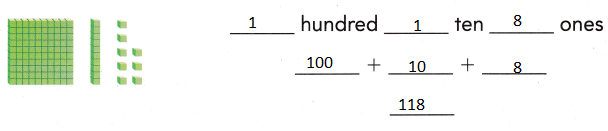Explanation:
Total number is blocks are 118
So we can write as 1 hundred 1 ten and 8 ones

Question 2.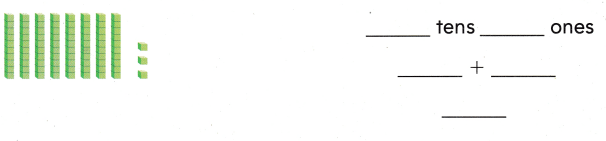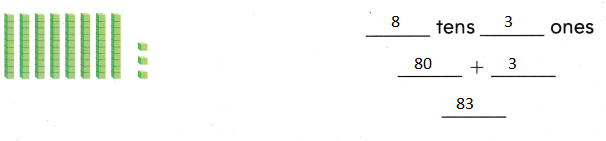Explanation:
Total number is blocks are 83
So we can write as 8 tens and 3 ones

Problem Solving

Use the model. Write the number three different ways.

Question 3.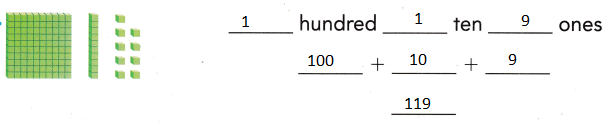Explanation:
119 is a three-digit number.
The 1 in 119 means 1 hundred.
The 1 in 119 means 1 ten.
The 9 in 119 means 9 ones.

Draw a quick picture to show the number. Write the number three different ways.

Question 4.
H.O.T. Dora has 9 tens. Tim has 23 ones. They put all their tens and ones together. What number did they make?_____ hundred ___ ten __ ones
___ + ___ + _____
_______
1 hundred 1 ten 3 ones
100 + 10 + 3= 113
Explanation:
Dora has 9 tens.
Tim has 23 ones = 2 tens and 3 ones
9 tens + 2 tens + 3 ones = 11 tens + 3 ones = 113

Question 5.
H.O.T. Multi-Step Peter has 10 tens. Maria has 1 ten. They put all their tens together. Olivia put 6 ones with all the tens. What number did they make?
_____ hundred ___ ten __ ones
___ + ___ + _____
_______
1 hundred 1 ten 6 ones
100 + 10 + 6 = 116
Explanation:
Peter has 10 tens. Maria has 1 ten.
10  tens + 1  tens = 11 tens
Olivia put 6 ones with all the tens.
11 tens + 6 ones = 116

Question 6.
Tyler counts blocks. There are 10 tens and 4 ones. How many blocks did he count?
(A) 14
(B) 104
(C) 140
Explanation:
10 tens  + 4 ones = 100 + 4 = 104

Question 7.
Use Tools Ginger made this model. What number does her model show?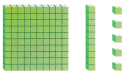(A) 115
(B) 15
(C) 105
Explanation:
1 hundred + 1 ten + 5 ones = 100 + 10 +5 = 115

Question 8.
Which shows the same number?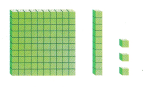(A) 1 hundred 3 ones
(B) 10 tens 13 ones
(C) 10 tens 3 ones
Explanation:
1 hundred + 1 ten + 3 ones = 10 tens + 10 ones + 3 ones = 10 tens + 13 ones

Question 9.
Texas Test Prep Which shows the same number?(A) 1 hundred 1 ten 3 ones
(B) 1 hundred 2 ones
(C) 1 hundred 1 ten 1 one
Explanation:
11 tens + 3 ones =10 tens + 1 ten + 3 ones = 1 hundred + 1 ten + 3 ones

### Texas Go Math Grade 1 Lesson 1.8 Homework and Practice Answer Key

Use the model. Write the number three different ways.

Question 1.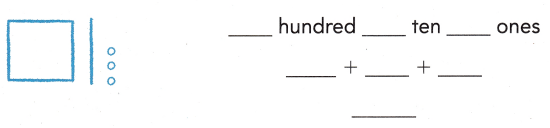Explanation:
Square = 1 hundred
Line = 1 ten
circle = 1 ones
1 hundred + 1 ten + 3 ones = 100 + 10 + 3 = 113

Question 2.Explanation:
9 tens + 6 ones = 90 + 6 = 96

Problem Solving

Choose a way to solve. Draw or write to explain.

Question 3.
Multi-Step Lev has 9 tens. Jim has 1 ten. They put all their tens together. Dawn puts 9 ones with the tens. What number did they make?
____ hundred ___ tens ___ ones
______ + _____ + _____
_____
10 tens 9 ones
100 + 9 = 109
Explanation:
Lev has 9 tens. = 90
Jim has 1 ten. = 10
90 + 10 = 100
Dawn puts 9 ones with the tens.
100 + 9 = 109

Lesson Check

Question 4.
Ali bounces a ball while Tim counts. Tim counts 11 tens and 2 ones. How many bounces did Tim count?
(A) 112
(B) 102
(C) 120
Explanation:
Tim counts 11 tens and 2 ones = 110 + 2 =112

Question 5.
Tim uses blocks to count the bounces. What number does the model show?(A) 116
(B) 106
(C) 16
Explanation:
1 hundred + 6 ones = 100 + 6 = 106

Question 6.
Which shows the same number?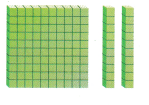(A) 1 hundred 2 ones
(B) 11 tens 20 ones
(C) 10 tens 20 ones
Explanation:
1 hundred = 10 tens
2 tens = 20 ones
So 10 tens 20 ones

Question 7.
Which shows the same number?